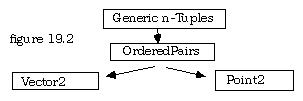## 19.11 Extended Example--Points and Vectors

The module Vectors in section 7.11 and the module Points in section 6.9 share some basic concepts in common. These two data types could be implemented as classes with the latter as the superclass as shown below.

```DEFINITION MODULE PointClassA;

TRACED CLASS Points;
REVEAL assignR, firstCoord, secondCoord, abs, arg, assignP, reflectX, reflectY,
reflect0, reflect45, scale, rotate, translate;
TYPE
Point = ARRAY [1 .. 2] OF REAL;
VAR
point : Point;

PROCEDURE assignR (x, y : REAL);
PROCEDURE firstCoord () : REAL;
PROCEDURE secondCoord () : REAL;
PROCEDURE abs () : REAL;
PROCEDURE arg () : REAL;
PROCEDURE assignP (abs, arg : REAL);
PROCEDURE reflectX;
PROCEDURE reflectY;
PROCEDURE reflect0;
PROCEDURE reflect45;
PROCEDURE scale (scaleFactor : REAL);
PROCEDURE rotate (rotAngle : REAL);
PROCEDURE translate (deltaX, deltaY : REAL);
END Points;

END PointClassA.```

A few names have been changed here to make them more generic for subclasses. The reader should compare this with the original and note the simplicity of the definition when the parameter of type Point can be left out because the procedure acts only on the variable point that is its attribute component. When moving from here to subclass Vectors, it seems appropriate to have a new type name, and to remove all functional duplicates. This results in an even simpler interface:

```DEFINITION MODULE VectorClassA;
FROM PointClassA IMPORT
Points;
TRACED CLASS Vectors;
INHERIT Points;
TYPE
Vector = Point;
PROCEDURE neg;
PROCEDURE sub (v : Vector);
PROCEDURE dotProduct (v : Vector) : REAL;
END Vectors;

END VectorClassA.```

However, this design leaves something to be desired, because reflections and rotations, while relevant to points are not relevant to vectors. Moreover, while one might scale a point, it is thought of as a multiplication with respect to vectors. It is better design to come up with a base or superclass that has only the bare essentials that are common, and then derive both Points and Vectors from this. Such a more basic concept is the idea of an ordered pair, or more generally, of an ordered n-tuple. This base class can have in it only the facilities for working with such tuples, and let both Points and Vectors define their own components. Figure 19.2 illustrates the relationship among the classes:Indeed, in the design of this suite of classes, the base class or first superclass is a generic module.

```GENERIC DEFINITION MODULE TupleClass (length : CARDINAL);
(* base class for specific n-tuples
R. Sutcliffe 1998 10 02 *)

TRACED CLASS Tuples;
REVEAL assignCoord, fetchCoord, abs;
CONST
len = length; (* for inheritors *)
VAR
coords : ARRAY [1 .. len] OF REAL;

PROCEDURE assignCoord (coordNum : CARDINAL; value : REAL);
PROCEDURE fetchCoord (coordNum : CARDINAL) : REAL;
PROCEDURE abs () : REAL;
END Tuples;

END TupleClass.```

To work with two dimensional vectors and points in a plane, this has to be refined by:

```DEFINITION MODULE OrderedPair = TupleClass (2);
(* produce a 2-dimensional base class of tuples for
corresponding vectors and points
R. Sutcliffe 1998 09 28 *)
END OrderedPair.```

This in turn can be subclassed on the one hand to work with points in the plane.

```DEFINITION MODULE Point2Class;
(* subclasses OrderedPairs for the geometric notions of points
R. Sutcliffe  1998 10 02 *)

FROM OrderedPair IMPORT
Tuples;
TRACED CLASS Points;
INHERIT Tuples;
REVEAL  reflectInAxis, reflect0, reflect45, scale, rotate, translate;

PROCEDURE arg () : REAL;
PROCEDURE reflectInAxis (n : CARDINAL);
PROCEDURE reflect0;
PROCEDURE reflect45;
PROCEDURE scale (scaleFactor : REAL);
PROCEDURE rotate (rotAngle : REAL);
PROCEDURE translate (deltaX, deltaY : REAL);
END Points;

END Point2Class.```

On the other hand, it can be subclassed to work with two-dimensional vectors.

```DEFINITION MODULE Vector2Class;
(* subclasses OrderedPairs for vectors
R. Sutcliffe 1998 10 01 *)

FROM OrderedPair IMPORT
Tuples;
TRACED CLASS Vector;
INHERIT Tuples;
REVEAL abscissa, ordinate, neg, add, sub, dotProduct, arg;

PROCEDURE abscissa () : REAL;
PROCEDURE ordinate () : REAL;
PROCEDURE neg;
PROCEDURE sub (v : Vector);
PROCEDURE dotProduct (v : Vector) : REAL;
PROCEDURE arg () : REAL;

END Vector;

END Vector2Class.```

Observe that a method such as Add needs only to be passed the vector to add to SELF. In effect, such methods are messages to the object to add another vector to themselves. This is shown in some of the procedures of the partial implementation below.

```IMPLEMENTATION MODULE Vector2Class;
(* subclasses OrderedPairs for vectors
R. Sutcliffe 1998 10 01 *)

TRACED CLASS Vector;

PROCEDURE abscissa () : REAL;
BEGIN
RETURN coords ;
END abscissa;

PROCEDURE ordinate () : REAL;
BEGIN
RETURN coords ;
END ordinate;

PROCEDURE neg;
VAR
count : CARDINAL;
BEGIN
FOR count := 1 TO len
DO
coords [count] := - coords [count];
END;
END neg;

VAR
count : CARDINAL;
BEGIN
FOR count := 1 TO len
DO
coords [count] := coords [count] + v.coords [count];
END;

PROCEDURE sub (v : Vector);
END sub;

PROCEDURE dotProduct (v : Vector) : REAL;
VAR
count : CARDINAL;
sum : REAL;
BEGIN
sum := 0.0;
FOR count := 1 TO len
DO
sum := sum + coords [count] * v.coords [count];
END;
RETURN sum;
END dotProduct;

PROCEDURE arg () : REAL;
BEGIN
(* code to work out angle here. Specific to two-vectors. *)
END arg;

END Vector;

END Vector2Class.```

It should be evident to the reader that if complex numbers were not built in, they could be subclassed from ordered pairs as well, with new method components to handle such operations as multiplication and division.

Contents